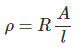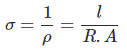# Electrical Properties of Engineering Materials

## Electrical Properties of Engineering Materials

To finalize the material for an engineering product / application, we should have the knowledge of Electrical properties of materials. The Electrical properties of a material are those which determine ability of material to be suitable for a particular Electrical Engineering Application. Some of the typical Electrical properties of engineering materials are listed below

• Resistivity
• Conductivity
• Temperature coefficient of Resistance
• Permittivity
• Thermoelectricity

## Resistivity

It the property of material which resists the flow of electric current through material. It is the reciprocal of conductivity.It is dented by ‘ρ’. Resistivity of a material of a conductor can be determined as below Where, ‘R’ is the resistance of conductor in Ω ‘A’ is the cross sectional area of conductor in m2 ‘l’ is the length of the conductor in meter SI unit of resistivity of is Ω¦-meter.
Resistivity of some materials is listed below,
 Sl. No. Element Resistivity at 20°C in Ω – m 1 Silver 1.59 × 10-8 2 Copper 1.7 × 10-8 3 Gold 2.44 × 10-8 4 Aluminum 2.82 × 10-8 5 Tungsten 5.6 × 10-8

## Conductivity

It is the property of material with allow the flow of electric current through material. It is a parameter which indicates that how easily electric current can flow through the material. It is denoted by ‘σ’. Conductivity of material is the reciprocal of resistivity. Conductivity of material can be determined by,Its SI unit is 1/(Ω-meter) or ℧/meter.

## Temperature Coefficient of Resistance

The temperature coefficient of resistance of a material indicates the change in resistance of material with change in temperature. Resistance of conductor changes with change of temperature. The rise in resistance of a material with rise in temperature depends on following things,
1. R2 – R1 ∝ R1
2. R2 – R1 ∝ t2 – t1
3. Property of material of conductor.
Where, R1 is the resistance of conductor at temperature of t1°C and R2 is the resistance of conductor at temperature of t2°C. Hence, from above, R2 – R1 ∝ R1 (t2 – t1) Or, R2 – R11 R1(t2 – t1) ⇒ R2=R1 [1+α1 (t2 – t1)] Where, α1 is temperature coefficient of resistance of material at temperature of t1°C. Its unit is /°C. Temperature coefficient of resistance of material is also depends on temperature. Temperature coefficient of some materials are listed below

 Sl. No. Element Temperature Coefficient of Resistance in /°C 1 Manganin 0.00002 2 Constantan 0.00017 3 Nichrome 0.0004 4 Mercury 0.0009 5 Silver 0.0038

## Thermoelectricity

If the junction, formed by joining to two metals, is heated, a small voltage in the range of millivolt is produced. This effect is called thermoelectricity or thermoelectric effect. This effect forms the basis of operation of thermocouples and some temperature based transducers. This effect can be used to generate electricity, to measure the temperature and to measure the change is temperature of objects.

## Dielectric Strength

It is the property of material which indicates the ability of material to withstand at high voltages. Generally it is specified for insulating material to represent their operating voltage. A material having high dielectric strength can withstand at high voltages. Generally, it is represented in the unit of KV/cm. Dielectric strength of some insulating materials are listed below
 Sl. No. Material Dielectric Strength [KV(max.)/cm] 1 Air 30 2 Porcelain 80 3 Paraffin Wax 120 4 Transformer oil 160

## One thought on “Electrical Properties of Engineering Materials”

1.sheik says:

Very useful news